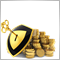• 概要
• レビュー (1)
• コメント (15)

# Triple Moving Average tfmt4

Using three moving averages, this Expert Advisor opens positions when all 3 MAs are moving in the same direction. As the fastest moving average crosses back, the EA exits the positions. You can choose moving averages with fewer bars to catch shorter term trends or lengthen the number of bars in the moving averages to catch longer term trends.

Note: The default input values are not optimized. Demo the EA and adjust the inputs to find the optimized combination for your risk tolerance and to maximize profitability. Trend Following systems are designed around long term probabilities. Although Trend Following systems have lower win rates, profitability comes from large trends as Trend Following cuts losses short and lets winners run. Test on a portfolio of symbols as profits from trending symbols will offset the small losses and provide profits when other symbols are not trending.

Entries and Pyramiding:

Entries occur when the fastest moving average is outside the middle moving average and the middle moving average is outside of the slowest moving average. See the screenshot for an example. If you set the Max Unit variable above 1, additional entries will occur and pyramid in ATR increments specified by the ATR between Pyramids variable.

Exits:

Exits occur when the fastest moving average crosses back across the middle moving average. The exit uses the closed moving averages of the previous bar.

Position Sizing and Stops:

This EA calculates the position sizing using the Percent Volatility method which is directly tied to the stop. The stop uses the ATR_Periods and Stop_Range_ATR inputs to calculate the ATR and then multiply the two values to set the stop distance from the entry price. Stops are not coded into the position but this EA closes out the position if the price reaches the stop value. As additional units are added through pyramiding, the stop moves to correspond to the latest entry price. Using the stop value, the Risk_Percent input, and your account information (tick size, lot size, digits, etc.), the position sizing uses the monetary value of the distance from entry to stop and keeps the number of lots limited to the percentage you specify. This allows every symbol, price, volatility to be treated equally. As your account size changes through profits or drawdowns, the position sizing will account for the change.

Inputs:

• Short_MA: The number of bars used to create the fastest moving (least bars) simple moving average.
• Middle_MA: The number of bars used to create the middle moving simple moving average.
• Long_MA: The number of bars used to create the slowest moving (most bars) simple moving average.
• Risk_Percent: The percent risked per position if the price reaches the stop. Example: If you want 2% of your equity to be risked per position, enter 2 to this input.
• ATR_Periods: The number of bars to use in the ATR calculation.
• Stop_Range_ATR: This value will be multiplied by the ATR to determine where the stop will be from the entry price. Example: If you want your stop to be set at 2* ATR from the price, enter 2 to this input.
• Max_Units: The maximum number of entries (including the initial entry) as the position gains profits and the EA adds pyramid positions.
• ATR_between_Pyramids: This value will be multiplied by the ATR to use for calculating when to add the next position through pyramiding. Example: Set this to 1.5 and the next pyramid position would be added when the price reaches your entry plus (1.5 * ATR) for long positions or entry minus (1.5 * ATR) for short positions.
• Slippage: Amount of allowable slippage when entering position.
• Reduction_Percent: Enter an amount by which to reduce your equity for the position sizing calculation. Example: If you are in a drawdown period you can enter 20 to this input and the position size will be 20% less than without the reduction. The position sizing calculation would treat your equity as 80% of what it really is to lower your risk until the drawdown is over.20974
2018.11.03 12:28

Good